# Foundation Fieldbus Pressure Transmitter Configuration

#### Foundation Fieldbus Pressure Transmitter Configuration

FF Pressure transmitter basic configuration parameters are L_Type, XD_Scale, OUT_Scale and Channel.

These parameters are configured in Analog Input (AI) Function block of FF transmitter.

The below problems shows the pressure transmitter configurations for different cases.

#### A pressure transmitter with a range of 0 – 100 psi. How to configure a foundation fieldbus pressure transmitter ?

Analog Input function block configuration for a typical pressure transmitter as per below table :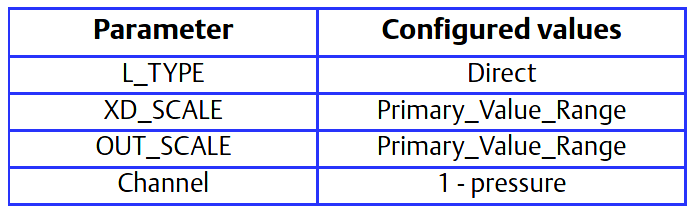Here Primary_Value_Range : 0 to 100

#### The level of an open tank is to be measured using a pressure tap at the bottom of the tank. The maximum level of the tank is 16 ft. The liquid in the tank has a density that makes the maximum level correspond to a pressure of 7.0 psi at the pressure tap (see below Figure).The table below lists the appropriate configuration settings.

Analog Input function block configuration for a pressure transmitter used in level measurement (problem #1).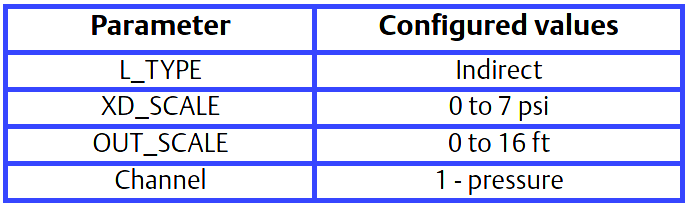Output calculation for Problem 2 :

When the L_Type is configured as Indirect, the OUT parameter is calculated as: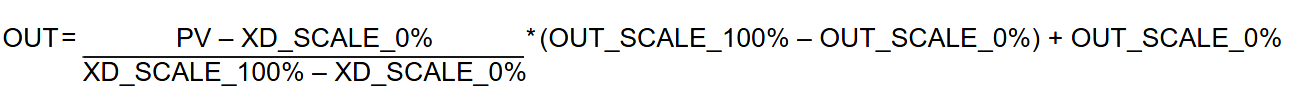OUT =  [ (PV – XD_SCALE_0% ) / (XD_SCALE_100% – XD_SCALE_0%) ] x ( (OUT_SCALE_100% – OUT_SCALE_0%) + OUT_SCALE_0%)

In this example, when PV is 5 psi, then the OUT parameter will be calculated as follows:

OUT =  [ ( 5 psi – 0 psi ) / (7 psi – 0 psi) ] x ((16 ft – 0 ft) + 0 ft ) =  11.43 ft

#### The transmitter in problem #3 is installed below the tank in a position where the liquid column in the impulse line, with an empty tank, is equivalent to 2.0 psi (see Below Figure ).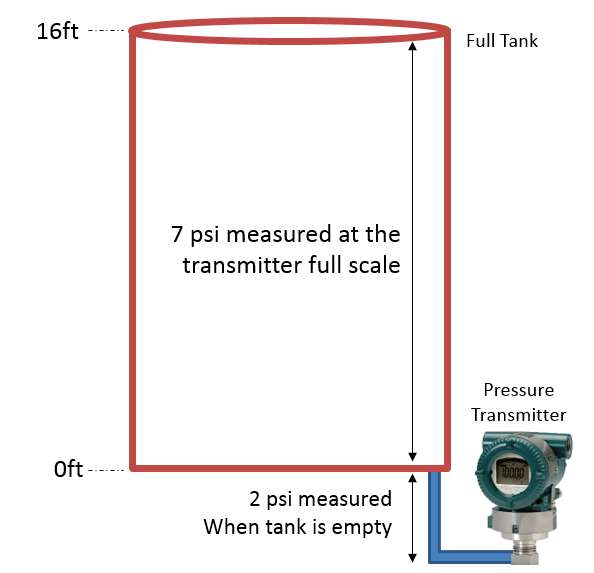Solution to Problem #3

The table below lists the appropriate configuration settings.

Analog Input function block configuration for a pressure transmitter used in level measurement (Problem #3).In this example, when the PV is 4 psi, OUT will be calculated as follows:

OUT = [ (4 psi – 2 psi ) / (9 psi – 2 psi) ] x (  (16 ft. – 0 ft.) + 0 ft.) = 4.57 ft.

#### Problem 4 :-

The liquid flow in a line is to be measured using the differential pressure across an orifice plate in the line. Based on the orifice specification sheet, the differential pressure transmitter was calibrated for 0 to 20 inH2O for a flow of 0 to 800 gal/min.

Solution

The table below lists the appropriate configuration settings.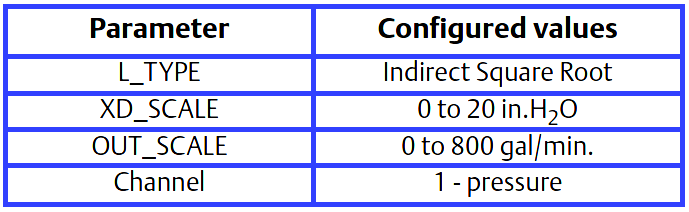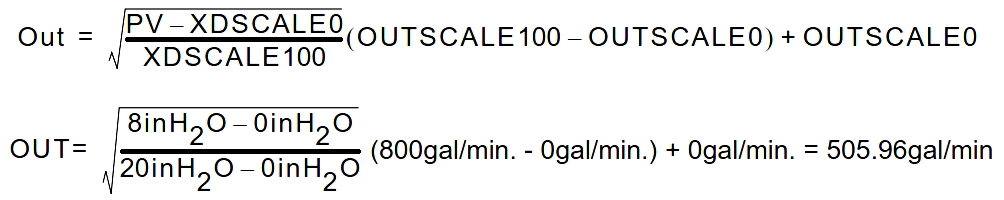Reference : Rosemount Pressure Transmitter Manual

Be the first to get exclusive content straight to your email.
We promise not to spam you. You can unsubscribe at any time.

### 3 thoughts on “Foundation Fieldbus Pressure Transmitter Configuration”

1.Excellent document to lean from Pressure Transmitter

2.•